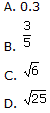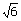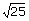Definition Of Irrational Number

Irrational numbers are real numbers that cannot be expressed as fractions, terminating decimals, or repeating decimals.

Example of Irrational Numberare few examples of irrational numbers.

Solved Example on Irrational Number

Ques: dentify the irrational number.

Choices:Step 1: The value ofis 2.4494897427831780981972840747059. . .
Step 2: The value ofis 5.
Step 4: Among the choices given, onlyis an irrational number.